# Robotics, mathematical problems in

(diff) ← Older revision | Latest revision (diff) | Newer revision → (diff)

Robotics studies deal with the design of autonomous and semi-autonomous machines intended to perform complex tasks in a wide range of applications, from assembly in industrial settings to waste material disposal, to exploration and exploitation of resources in hazardous environments, as in space or underwater. Since a robotic manipulator is a complex mechatronic system, a strong interaction between a large number of different fields in applied mathematics, such as control and dynamical systems theory, theoretical mechanics, signal processing, informatics and artificial intelligence, is required to design a fully functional robotic work-cell. Generally speaking, the process of controlling a robotic manipulator to perform a given task requires three functional levels: sensing the environment through acquisition and processing of sensorial data, planning a strategy to execute the task that is able to integrate the acquired knowledge of the environment with the human supervision, and actuating the manipulator in such a way that the task is effectively performed despite the uncertainties intrinsic in the mathematical description of the interactions between the manipulator dynamics and the external environment. The three levels described above are generally referred to as sensing, planning and control. A strong degree of integration between sensing, planning and control is highly desirable in order to provide the robotic work-cell of autonomous capabilities, i.e., the capacity to utilize heterogenous pieces of sensor information to cope automatically with uncertainties or unpredicted events in a mutating environment, without the explicit intervention of the human operator. A key to the fulfillment of the above goal is the possibility of changing the specification of the task to be accomplished in real time as unexpected events occur, without the necessity to stop and/or replanning the entire strategy. Although a great deal of literature exists on the subject of motion planning (see [a2], [a1]), a general solution to the aforementioned problem is still (1999) to be found. One of the possible approaches recently investigated concerns so-called event-based motion analysis and planning, in which the formulation of the plan as a function of time is replaced by a function of a scalar auxiliary variable that is directly related to the motion of the manipulator in the task space, for example the distance travelled by the manipulator end effector along a given path (see [a9], [a10]). One of the major challenges in developing an integrated planning and control system for a robotic work-cell lies in the lack of a unified framework that bridges a link between different methodologies such as symbolic and geometric reasoning, discrete-event systems and time-based control. A system of this kind is called a hybrid system, since it combines discrete and continuous events. An important step towards the development of an analytical method for modeling and designing hybrid systems is to employ a max-plus algebra model of the manufacturing work-cell and expressing the relationship between discrete and continuous events by means of a unified auxiliary variable, as proposed in [a7].

The dynamics of a robotic manipulator are very well understood. A common way to derive the equations of motion is through the Euler–Lagrange equation (see [a6])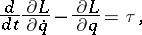(a1)

where the joint variables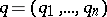are the generalized coordinates for the system, the Lagrangian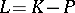is given by the difference between the kinetic energyand the potential energy, anddenotes the generalized forces that actuate the robot joints. The basic dynamic model that can be obtained by (a1) is given by(a2)

whereis the inertia tensor of the manipulator,contains centrifugal and Coriolis terms,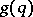is the gravity force exerted on the manipulator links, and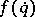is the joint friction. Model (a2) can be extended to incorporate actuator inertia, actuator dynamics and joint elasticity. A great number of control strategies has been investigated from 1975 onwards in order to provide accurate motion control. As (a2) is an inherently non-linear and strongly coupled dynamical system, non-linear control techniques are prominent in robotics. The most widely used are based on feedback linearization, that is, cancellation of the known non-linearities that are replaced by a linear, time invariant and decoupled model, for which classical control design techniques can be employed. In order to ensure insensitivity to model uncertainties, parameter variations and external disturbances, adaptive and robust control schemes are often employed in conjunction with the non-linear decoupling feedback. An excellent exposition of the state of the art in robot control is given in [a5] and [a4].

While model (a2) and its aforementioned extensions capture the intrinsic dynamics of the manipulator, almost-all applications involve some sort of interaction with the environment, which include the exertion of forces and torques on the end effector. In this case, even the finest position control is not sufficient for a successful accomplishment of a task, as force feedback is required to control the interaction between the end effector and the manipulated object. The interaction between the manipulator and the environment is performed in three phases, the free-motion phase, the transition phase or impact phase, and the contact phase. During the contact phase, the dynamical model (a2) is replaced by(a3)

where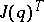is the transpose of the manipulator Jacobian, relating velocity in the joint space to velocity in the task space, andis the contact force in task space. While a different number of approaches are available for dealing with the contact-phase, like impedance control and hybrid force-position control (see [a5] and [a4]), control during the transition phase is a challenging subject in the literature of force control, due to the non-zero impact velocity and the unknown non-linear relationship between reaction forces and deformation. The manipulator may leave the contact surface after impact, and the occurrence of instability is often observed. Early approaches to the solution of the transition-phase control problem make use of impedance control and discontinuous control schemes. However, these methods have the disadvantage of requiring an accurate model of the environment parameters, such as stiffness and surface location, an unrealistic situation when dealing with different or unknown materials. In [a8] it is shown how positive acceleration feedback can be employed in conjunction with a switching control strategy to reduce bouncing caused by overshooting of the transient force response during the impact. In this way, a stable contact transition can be guaranteed and the output force regulation can be achieved after contact is established without assuming the knowledge of the environment.

A situation that arises frequently in applications such as underwater, space or terrestrial mobile robots is the presence of more degrees of freedom than control inputs. As a consequence of the fact that the degrees of freedom cannot be independently actuated, one refers to this class of robotic systems as underactuated manipulators. Denoting by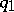the actuated generalized coordinates and by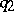the unactuated generalized coordinates, model (a2) reads as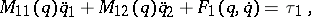(a4)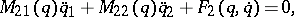where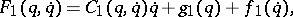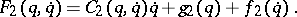As opposed to fully actuated robotic systems, very few results are sufficiently general to be applied to the entire class of underactuated systems, as the properties of model (a4) differ greatly from case to case. However, a common feature of underactuated manipulators is given by the fact that the motion must fulfill a differential constraint of the kind(a5)

which can be derived directly from (a4). If the differential constraint (a5) can not be integrated, that is, there is no twice continuously differentiable function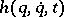which is constant along solutions of (a5), then one refers to (a4) as a second-order non-holonomic system. Identifying the non-holonomic behaviour of (a5) is crucial in determining the controllability and stabilizability properties of underactuated robotic systems. While it can be shown that, in general, an underactuated manipulator cannot be stabilized to an arbitrary equilibrium position by means of continuous time-invariant control laws (as it is the case for fully actuated manipulators), non-holonomy of the constraint (a5) is in many cases sufficient for the existence of smooth open-loop time-varying controls able to steer the system to any desired configuration. However, while those techniques are very well understood in the case of first-order (that is, kinematic) non-holonomic constraints (see [a3]), their extension to second-order constraints is currently (1999) the subject of major research efforts.

How to Cite This Entry:
Robotics, mathematical problems in. Encyclopedia of Mathematics. URL: http://encyclopediaofmath.org/index.php?title=Robotics,_mathematical_problems_in&oldid=13417
This article was adapted from an original article by Tzhy-Jong TarnAndrea Serrani (originator), which appeared in Encyclopedia of Mathematics - ISBN 1402006098. See original article